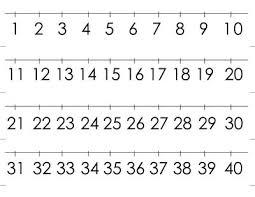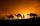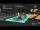# Number with ones

The first digit of the number is 1, if we move this digit to the end we get a 3 times higher number, which is the number?

Correct result:

n =  142857

#### Solution:We would be pleased if you find an error in the word problem, spelling mistakes, or inaccuracies and send it to us. Thank you!

Showing 1 comment:Dr Math
prima cifra a unui numar este 1 daca mutam aceasta cifra la sfarsit obtinem un numar de 3 ori mai mare care este numarul?Tips to related online calculators
Do you have a linear equation or system of equations and looking for its solution? Or do you have quadratic equation?

## Next similar math problems:

• Here isHere is a data set (n=117) that has been sorted. 10.4 12.2 14.3 15.3 17.1 17.8 18 18.6 19.1 19.9 19.9 20.3 20.6 20.7 20.7 21.2 21.3 22 22.1 22.3 22.8 23 23 23.1 23.5 24.1 24.1 24.4 24.5 24.8 24.9 25.4 25.4 25.5 25.7 25.9 26 26.1 26.2 26.7 26.8 27.5 27.6 2
• Solve 2Solve integer equation: a +b+c =30 a, b, c = can be odd natural number from this set (1,3,5,7,9,11,13,15)
• My fatherMy father has a big farm. 6/8 of it were planted with mango trees, 1/2 of the remainder are guava trees and the rest 10 trees are santol trees. What is the number of all trees?
• Self-counting machineThe self-counting machine works exactly like a calculator. The innkeeper wanted to add several three-digit natural numbers on his own. On the first attempt, he got the result in 2224. To check, he added these numbers again and he got 2198. Therefore, he a
• PoolIf water flows into the pool by two inlets, fill the whole for 19 hours. The first inlet filled pool 5 hour longer than the second. How long pool take to fill with two inlets separately?
• Unknown number 10Number first increased by 30%, then by 1/5. What percentage we've increased the original number?
• Unknown number 5I think unknown number. If we enlarge it five times then subtract 3 and result decreases by 75% we get the number by one greater than the number I think. What number am I thinking?
• The HotelThe Holiday Hotel has the same number of rooms on each floor. Rooms are numbered with natural numbers sequentially from the first floor, no number is omitted, and each room has a different number. Three tourists arrived at the hotel. The first one was in
• CamelCamel owner wants to get out of the city into oasis. He bought 3000 bananas, which wants to sell in oasis. However oasis from the city divided 1,000 kilometers of desert. Camel can carry at one time up to 1000 bananas, and for every kilometer traveled, ea
• DiophantusWe know little about this Greek mathematician from Alexandria, except that he lived around 3rd century A. D. Thanks to an admirer of his, who described his life through an algebraic riddle, we know at least something about his life. Diophantus's youth las
• Two-digit numberDigit sum of thinking two-digit natural number is 11. When it exchanging a sequence of digits, given a number which is 27 less than the thinking number. Find out which number I think.The voltage station is every day changing the master password, which consists of three letters. Code generation process does not change and is based on the following procedure: The following letters (A) to (I) correspond to different numbers from 1 to 9.Samuel wrote unknown number. Then he had add 200000 to the number and the result multiply by three. When it calculated he was surprised, because the result would have received anyway, if write digit to the end of original number. Find unknown number.The sum of three numbers from which second number is 20% smaller than the first number and the third number is 25% smaller than the second number is 96. Determine this numbers.PIN on Michael credit card is a four-digit number. Michael told this to his friend: • It is a prime number - that is, a number greater than 1, which is only divisible by number one and by itself. • The first digit is larger than the second. • The second dFrom the digits 1,2,3,4 we create the long integer number 123412341234. .. .. , which will have 962 digits. Is this number divisible by 6?In the competition, 10 contestants answer five questions, one question per round. Anyone who answers correctly will receive as many points as the number of competitors answered incorrectly in that round. One of the contestants after the contest said: We g